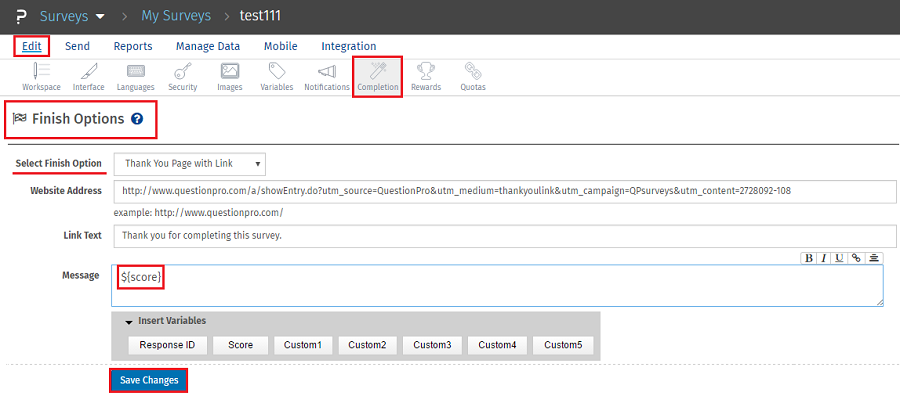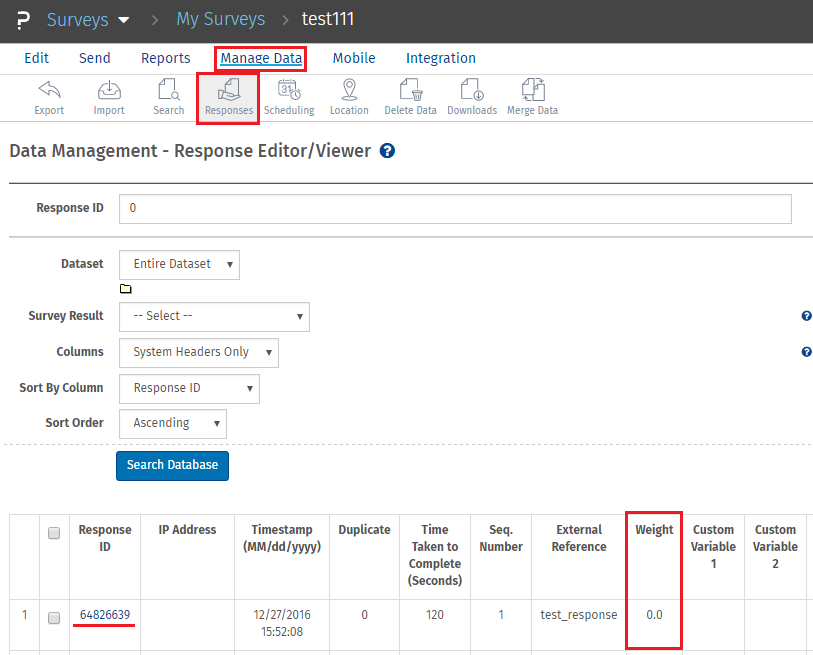# Scoring based on Custom Logic/Scripting

## Scoring Logic

Surveys can be used to compute scores / points in real-time. You can administer tests online and calculate scores and present them to the respondents immediately. Scoring Logic can be used for Tests, Quizes, etc.

## How to set up Scoring?

Consider the following example:

Q1: What is the Capital of the US?
1. New York
2. Seattle
3. Washington DC
4. Chicago
Q2: How many States are there in the US?
1. 20
2. 45
3. 50
4. 63
Q3: US Independence day is celebrated on:
1. 4th, June
2. 4th, July
3. 14th, July
4. 24th, Jan
Q4: The first President of the US was:
1. Washington, George
2. Adams, John
3. Jefferson, Thomas
4. Madison, James
Q5: The Statue of Liberty was gifted to US by:
1. Canada
2. France
3. England
4. Russia
In the above example survey the correct answers are as follows:
• Q1 = 3
• Q2 = 3
• Q3 = 2
• Q4 = 1
• Q5 = 2

## The Script for Scoring will be as follows:

This script can be used in a Custom Logic / Scoring Engine type question. For help on adding a Custom Logic/Scoring Engine type question please see help links below.

```
#set(\$tot=0)

#if (\${Q1} == 3)
#set(\$tot = \$tot + 1)
#end

#if (\${Q2} == 3)
#set(\$tot = \$tot + 1)
#end

#if (\${Q3} == 2)
#set(\$tot = \$tot + 1)
#end

#if (\${Q4} == 1)
#set(\$tot = \$tot + 1)
#end

#if (\${Q5} == 2)
#set(\$tot = \$tot + 1)
#end

#set(\$score = \$tot)

```

## How to calculate score for a Matrix Question?

Consider the above 2 matrix questions. Question codes for the matrix questions are Q1 and Q2 respectively. The score can be calculated as follows:

```
#set(\$score=\${Q1_1} + \${Q1_2} + \${Q1_3}) [This will calculate the score for the Matrix Q1]

#set(\$score=\${Q2_1} + \${Q2_2} + \${Q2_3}) [This will calculate the score for the Matrix Q2]

Score can be referenced directly using the Question Code.

Score for Matrix Q1: \$Q1
Score for Matrix Q2: \$Q2

The above example uses default values for the score. You can also set up custom score values for each individual option.
```

## Can I display the computed score to the end-user?

Yes - Use the \${score} variable in the "Thank You" page.

Login » Surveys » Edit » Completion » Finish Options## Can I (as the administrator) view the computed score for an individual?

Login » Surveys » Manage Data » Responses

Click on the Response ID for the Response. In the popup you can view the score in the Weight column.License & Access Options

This feature/tools described here are available with the following license(s) :

Team Edition

Unlimited Surveys, Questions

Advanced Toolset and Features

No Long Term Commitment

Was this article helpful?
Sorry about that
How can we improve it?